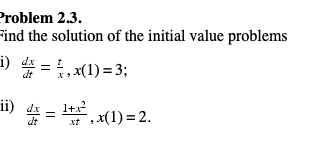# roblem 2.3. ind the solution of the initial value problems 1) dx dt x ii) 4x= x(1) = 2.

Question

Problem 2.3 i. and 2.3 iihelp_outlineImage Transcriptioncloseroblem 2.3. ind the solution of the initial value problems 1) dx dt x ii) 4x= x(1) = 2. fullscreen